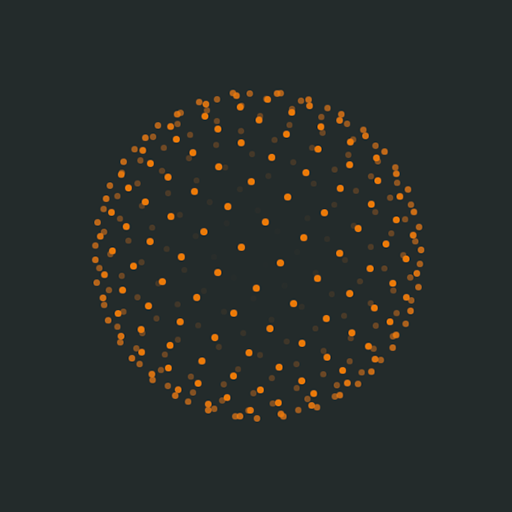# Fibonacci Sphere using CSS3 transformations

It has been some time since I don’t post anything, the project in which I’m working takes too much time from me. But this doesn’t prevent me to experiment a bit with the things that apasionate me.

I have wanted to test the performance of the main browsers using CSS3 transformations. In this little experiment, in each mouse movement, 300 DOM elements are transformed. I have used a Fibonacci calculation to create an sphere formed by equidistant points (known as Fibonacci Sphere). Using scale and opacity changing, I’ve tried to simulate certain depth effect. The performance is incredibly good in the main browsers, you can test it at the end of this article. The resulting code is the next one:

For this experiment, I’ve used the querySelectorAll Element method and CSS3 transformations. So, if you use Internet Explorer, you need to test it in a version greater than 9.

#### HTML

```<div id="canvas">

</div>
```

#### CSS (using autoprefixer)

```body, html{
height: 100%;
position: relative;
}

body{
background-color: #232B2B;
margin: 0;
}

#canvas{
height: 250px;
margin: 0 auto;
perspective: 2000px;
perspective-origin: center center;
position: relative;
top: 50%;
transform: translateY(-50%);
width: 250px;
}

.point{
background: #E87A24;
height: 4px;
position: absolute;
transform-style: preserve-3d;
width: 4px;
}
```

#### JavaScript

```var cant = 300;
var offset = 2 / cant;
var increment = Math.PI * (3 - Math.sqrt(5));
var canvas = document.getElementById("canvas");

var i;
var circle;

//---Build the elements
for(i = 0; i < cant; i++){

circle = document.createElement("div");
circle.className = "point";
circle.setAttribute("data-index", i);

canvas.appendChild(circle);

};

//---Apply transformations to points
function updatePoints(evt){

var x, y, z, r, a, scale, opacity, point, style;

var angle = (evt) ? (-evt.pageX / 4) * Math.PI / 180 : 0;

for(i = 0; i < cant; i++){

y = (i * offset - 1) + (offset / 2);
r = Math.sqrt(1 - Math.pow(y, 2));
a = ((i + 1) % cant) * increment + angle;
x = Math.cos(a) * r;
z = Math.sin(a) * r;

scale = Math.round(z * 20000) / 100;
opacity = (1 + z) / 1.5;

style = "translate3d(" + (125 + x * 100) + "px, " + (125 + y * 100) + "px, " + scale + "px)";

point = canvas.querySelectorAll("[data-index='" + i +"']");
point.style.WebkitTransform = style;
point.style.msTransform = style;
point.style.transform = style;
point.style.opacity = opacity;

}

}

//---Update the points at start
updatePoints();

//---Update the points on mouse move(3 votes, average: 5.00 Out Of 5)Fibonacci Sphere using CSS3 transformationscss3,experiments,Fibonacci,javascript,mathematics,sphere